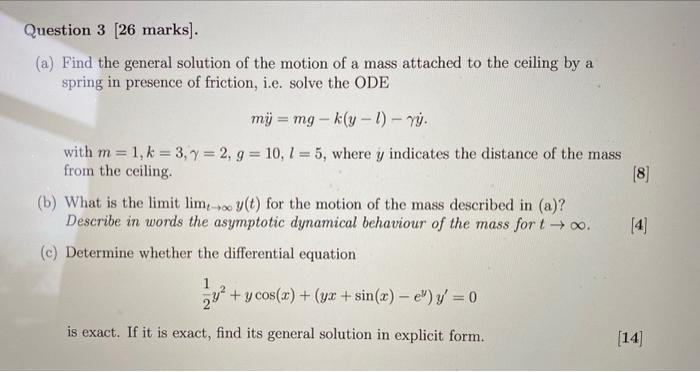# (Solved): handwritten solution only please  (a) Find the general solution of the motion of a mass attache ...(a) Find the general solution of the motion of a mass attached to the ceiling by a spring in presence of friction, i.e. solve the ODE $m \ddot{y}=m g-k(y-l)-\gamma \dot{y} .$ with $$m=1, k=3, \gamma=2, g=10, l=5$$, where $$y$$ indicates the distance of the mass from the ceiling. $$$$ (b) What is the limit $$\lim _{t \rightarrow \infty} y(t)$$ for the motion of the mass described in (a)? Describe in words the asymptotic dynamical behaviour of the mass for $$t \rightarrow \infty$$. $$$$ (c) Determine whether the differential equation $\frac{1}{2} y^{2}+y \cos (x)+\left(y x+\sin (x)-e^{y}\right) y^{\prime}=0$ is exact. If it is exact, find its general solution in explicit form. $$$$